## Integrating Clipped Spherical Harmonics

Laurent Belcour - Guofu Xie
University of Montreal
Christophe Hery - Mark Meyer
Pixar Animation Studio
Wojciech Jarosz
Dartmouth College
Derek Nowrouzezahrai
McGill University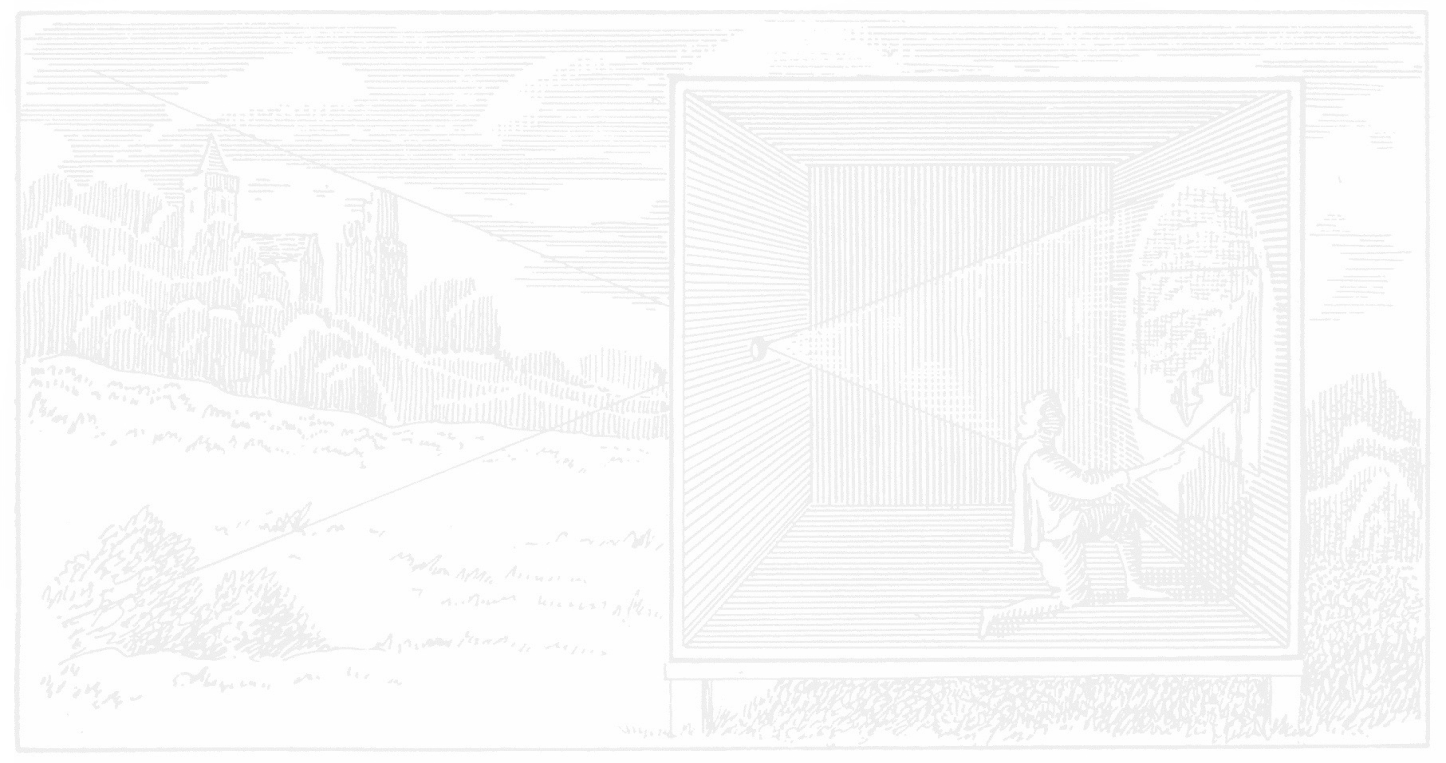## Motivation

• Spherical Integrals are common in rendering
• Importance sampling
• ...

• Analytical forms are not common

• But are needed by real-time rendering

## Motivation: BRDF Integration

• (Quasi) Monte-Carlo
• Approximative (contains error)
• Large number of samples
• Real-Time Rendering
• Sampling is out of the question
• Needs efficiency, allows approximate
• Analytical solutions are welcome
• Must include the light (spherical domain)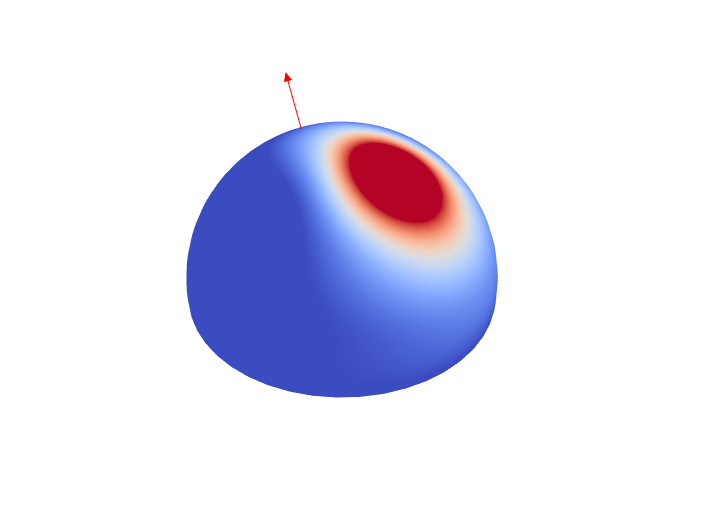BRDFlight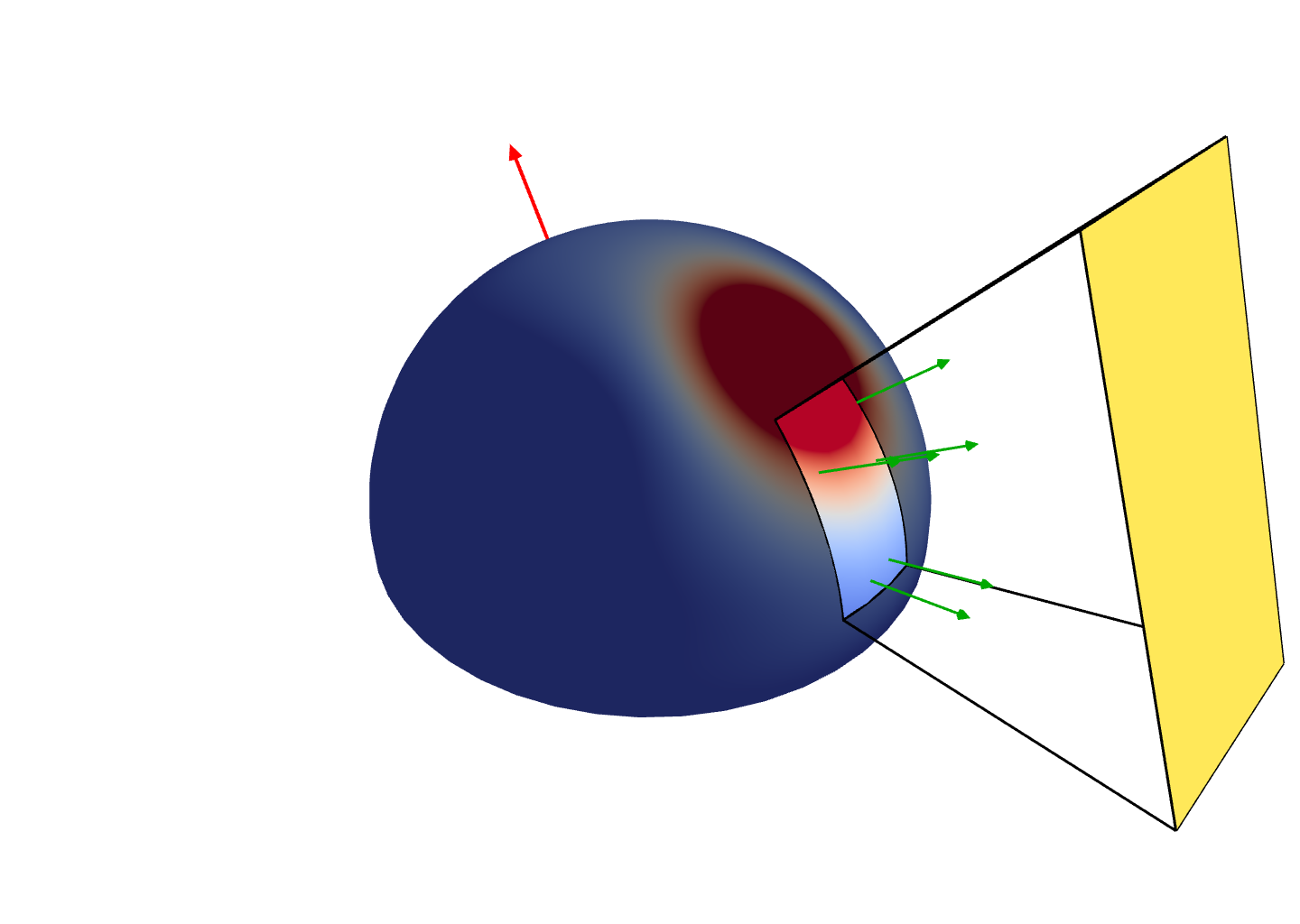## A Few Existing Methods

Polygonal lights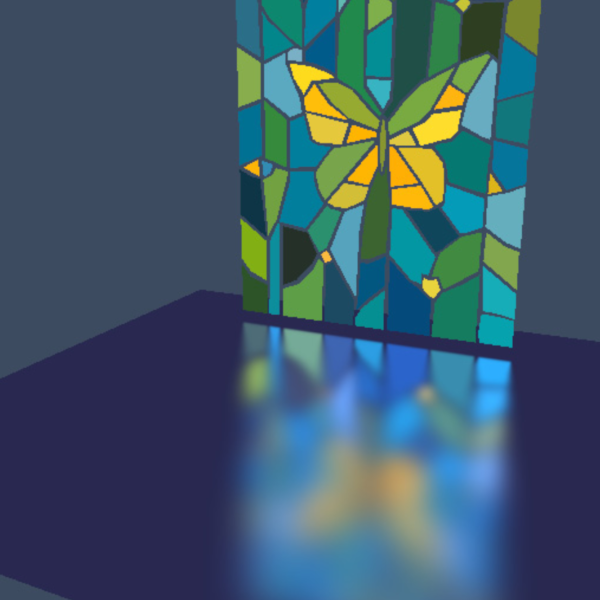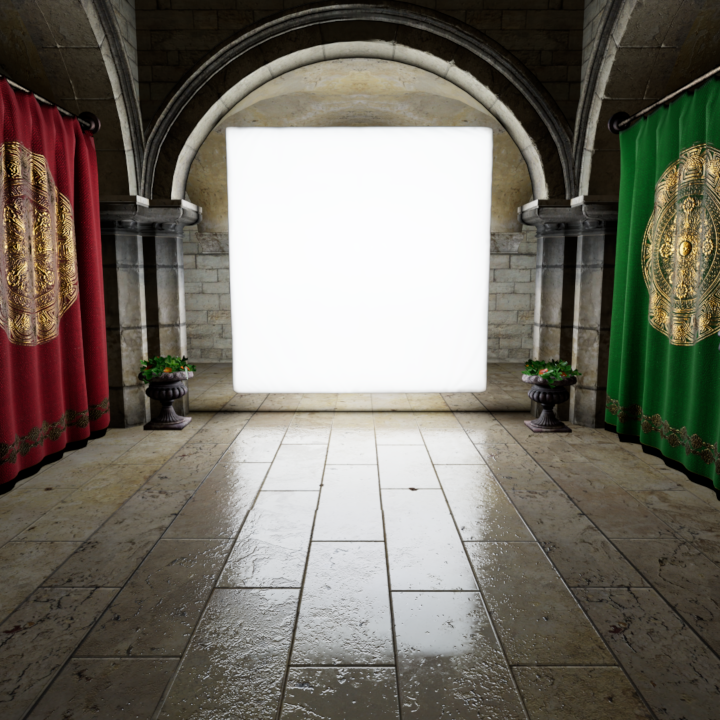[Arvo 1995]                     [Heitz et al. 2015]
Sphere lights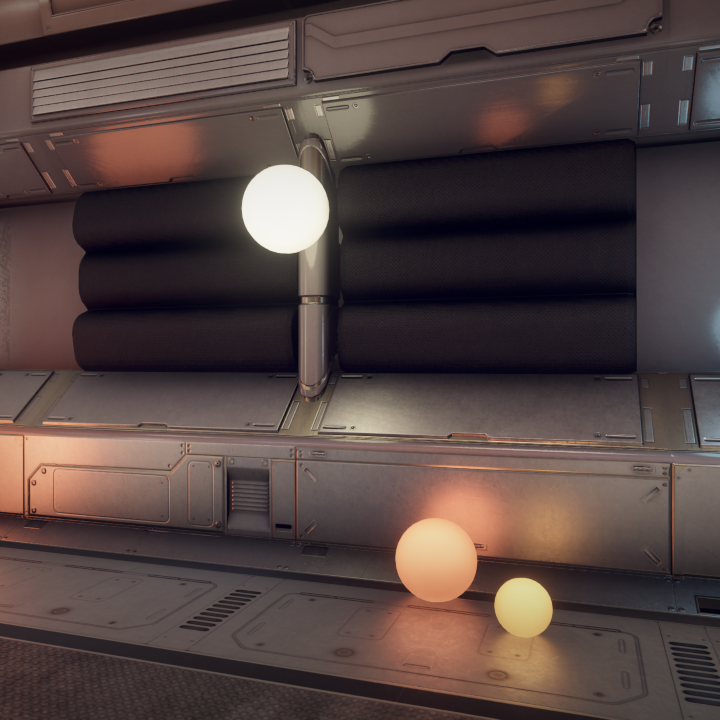[Dupuy et al. 2017]
Linear lights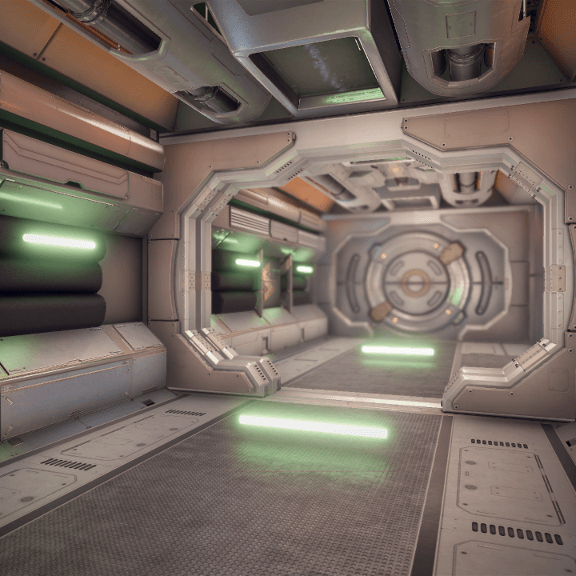[Heitz et al. 2017]
• But restricted to specific spherical functions

## Our Solution

• Integrate Spherical Harmonics expansions on spherical polygons
• Efficient algorithm
• scales well with higher order SH
• We incorporate such analytical solution

## Inspiration: Axial Moments

• Function of the dot product, $f(\omega) = \langle \omega \cdot \color{red}{\omega_i} \rangle^\color{green}{n}$
• Recursive integration [Arvo 1995]
• Linear with respect to the power $\color{green}{n}$
• Each step integrate another power cosine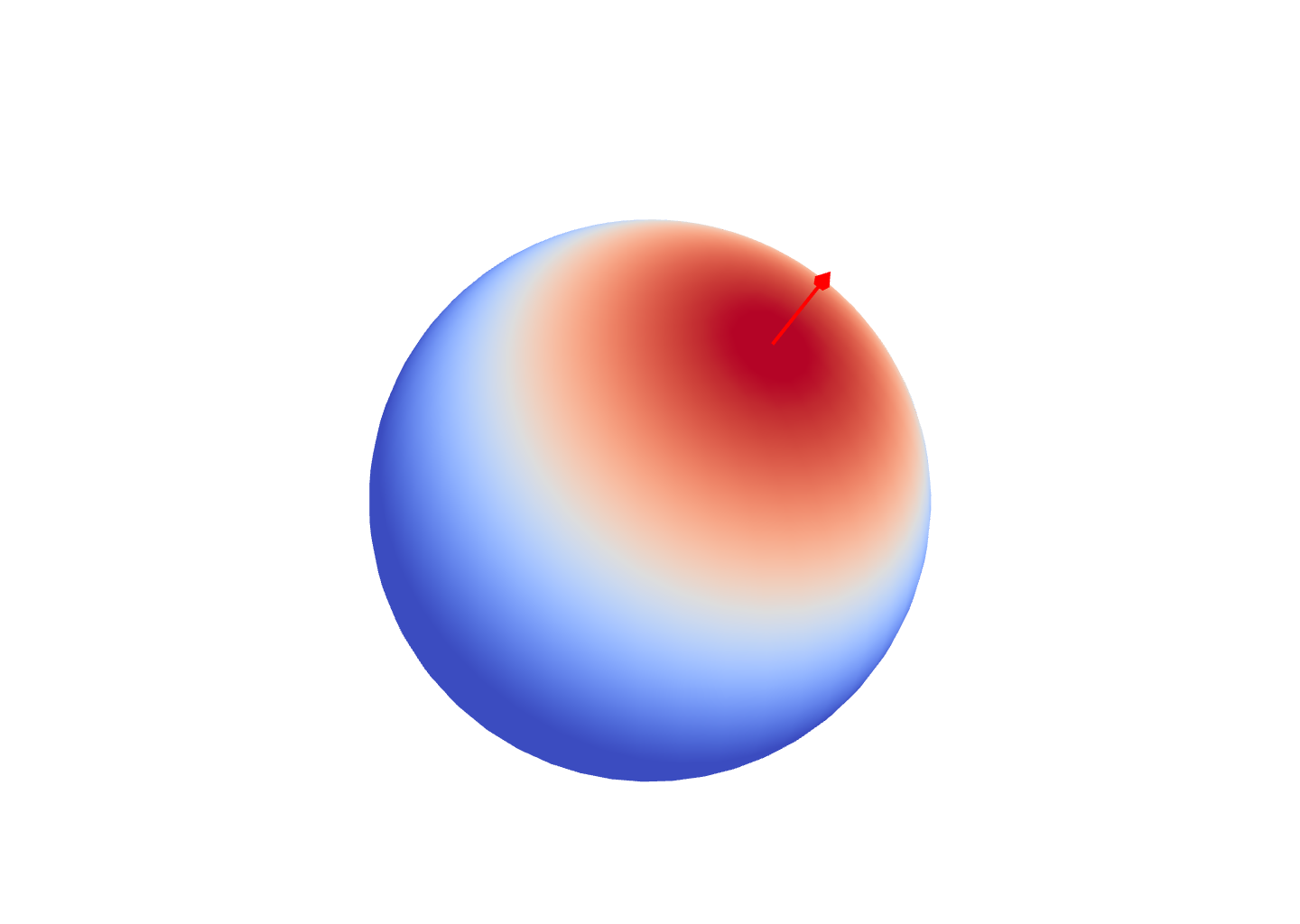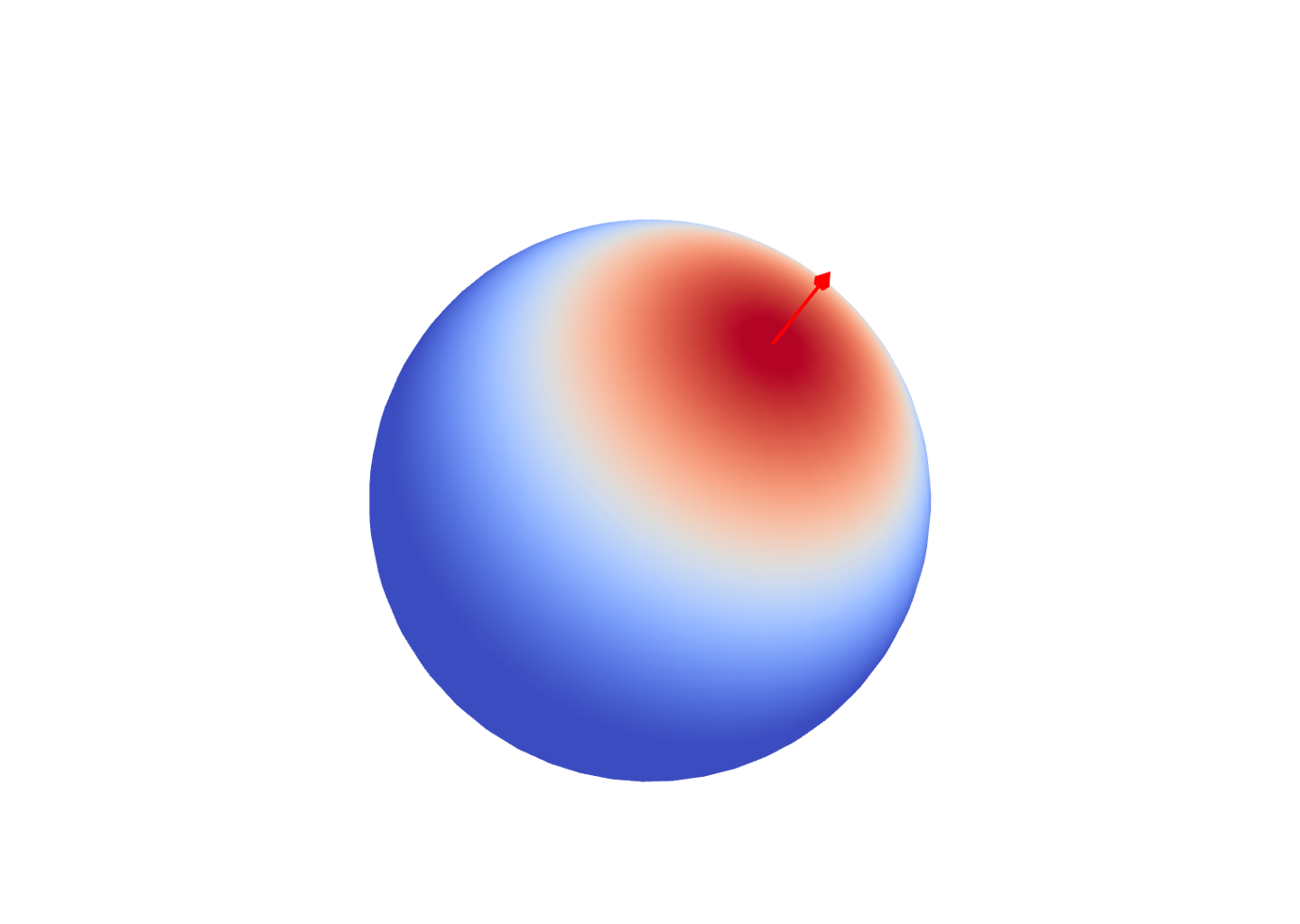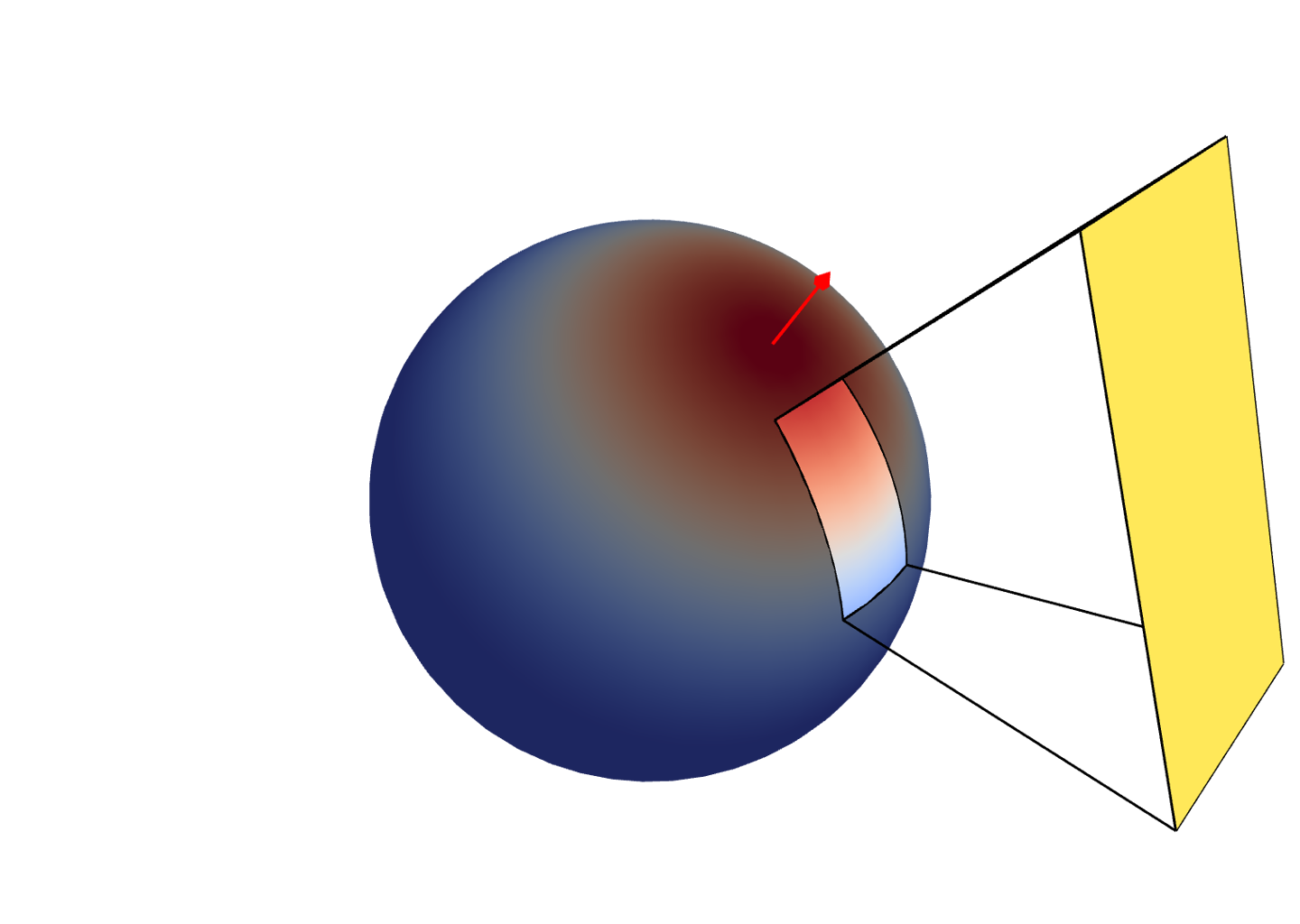$\color{red}{\omega_i}$
$\langle \omega \cdot \color{red}{\omega_i} \rangle^\color{green}{3}$
$\langle \omega \cdot \color{red}{\omega_i} \rangle^\color{green}{5}$

## Outline of Our Method

$$\int_\mathcal{P} f(\boldsymbol\omega) \mbox{d}\boldsymbol\omega$$
$$\sum f_{l,m} \int_\mathcal{P} y_{l}^{m}(\boldsymbol\omega) \mbox{d}\boldsymbol\omega$$
$$\sum c_{k,i} \int_\mathcal{P} \langle \boldsymbol\omega \cdot \boldsymbol\omega_i \rangle^{k} \mbox{d}\boldsymbol\omega$$
lobe sharing

## That's All Folks!

                            
/* Compute the integral of the SH decomposition with
* f coefficients over a spherical polygon poly.
*/
function ComputeIntegral(flm, poly) {

// Generate a set of vectors
basis = SharedDirections();

// Compute the conversion matrices
//   A converts SH to Zonals
//   P converts Zonals to Axials
A  = ZonalWeights(basis);
P  = AxialWeights(basis);
AP = A*P;

// Convert the Axial expansion
cpw = flm.transpose() * AP;

// Return the integral using Arvo's method
m = AxialMoments(poly, basis);
return cpw.dot(m);
}


$$\mathbf{f}^T \mathbf{y} = \sum_{l,m} \mathcal{\color{green}{f_{l,m}}} \left[ \int_{\mathcal{\color{darksalmon}{P}}} y_{l,m}(\omega) \right]$$$$\mathbf{c}^T = \mathbf{f}^T \times AP$$
$$\mathbf{f}^T \mathbf{y} = \underbrace{\mathbf{c}^T}_{\mathbf{f}^T \times AP} \times \mathbf{m}$$

## That's All Folks! (How Really?)

• Redudant computation in Arvo's method

• We improve performance by
• sharing axial moment directions
• sharing Arvo's recursive evaluation

• Details are in the paper

## Algorithmic Complexity

• Linear w.r.t. number of SH coefficients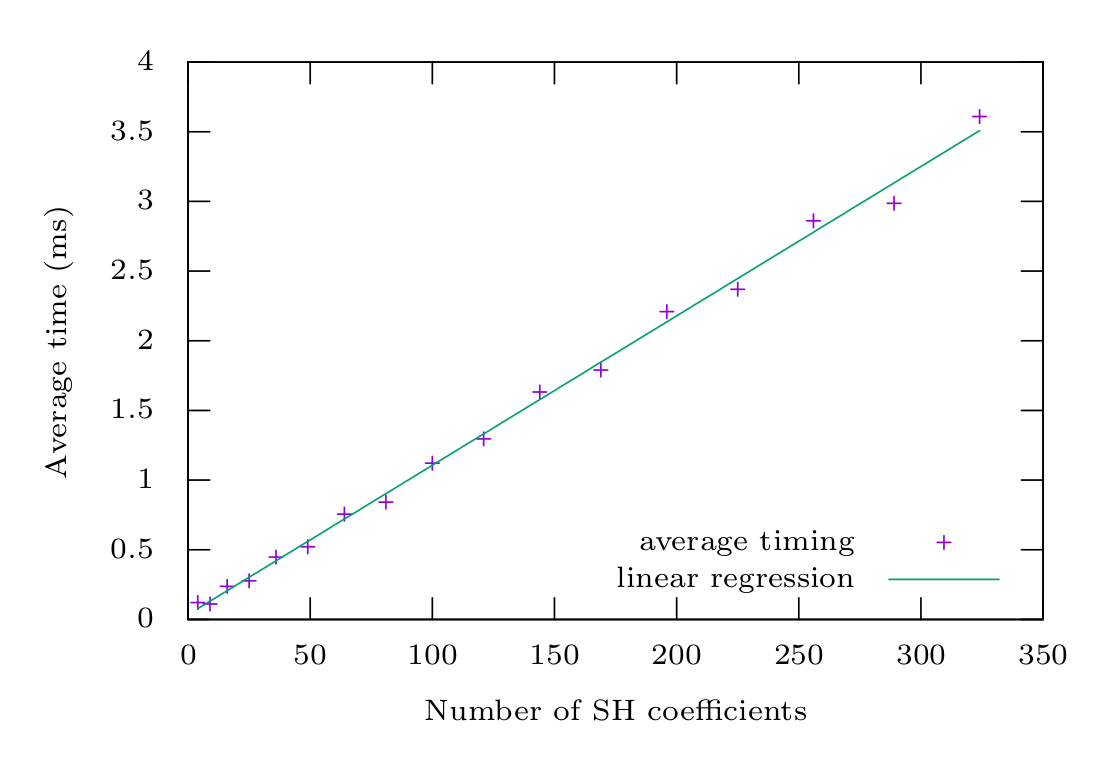## Applications

real-time rendering
importance sampling
hierarchichal warping
control variate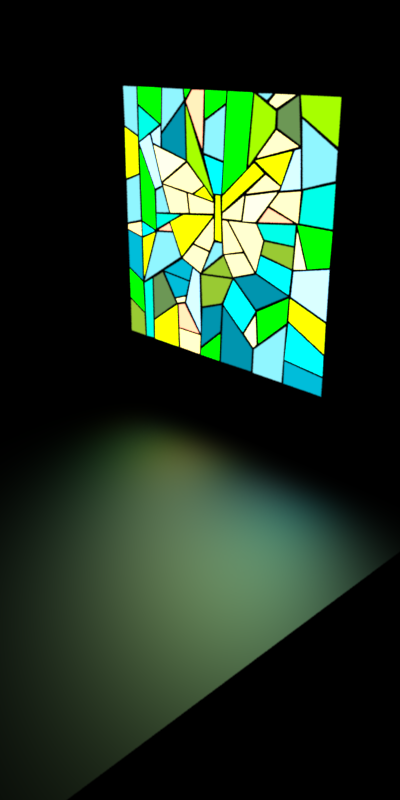Surface area light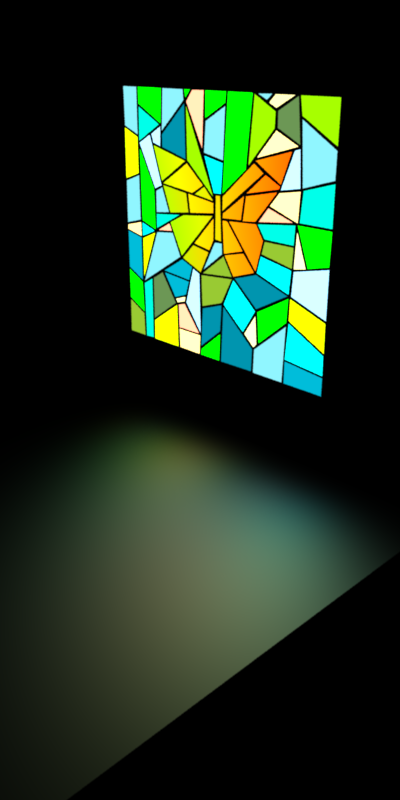Surface portal light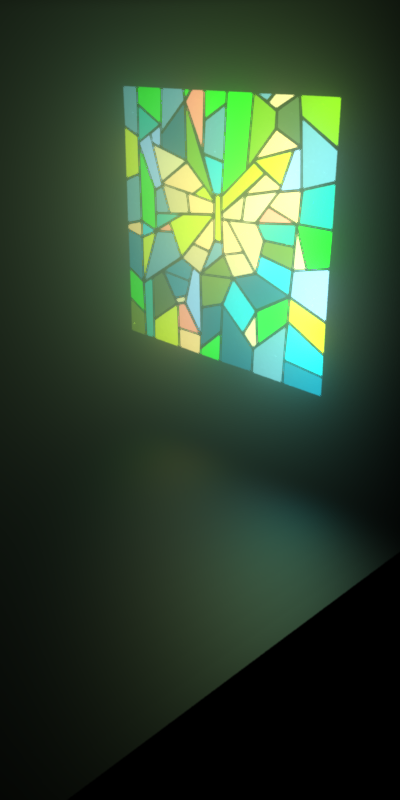Volume area light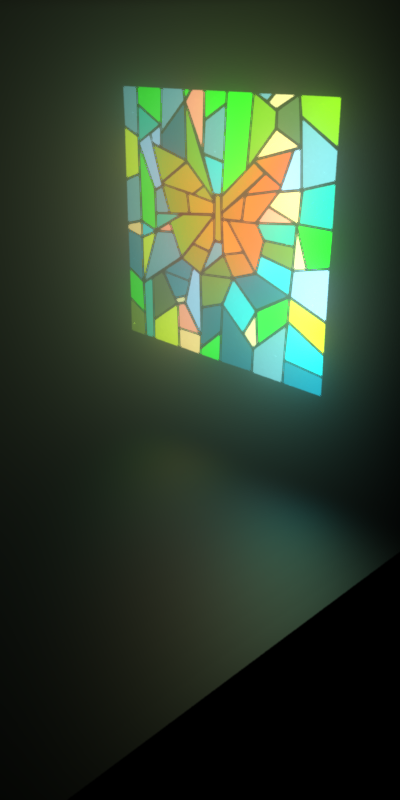Volume portal lightSurface area light
$$\color{green}{\rho(\boldsymbol{\omega}_i, \cdot}) \simeq \mathbf{f} = \mathbf{y}_i^T, \mbox{M} \quad \mbox{with} \, \, \mathbf{y}_i = y_{l,m}(\boldsymbol{\omega}_i)$$Surface portal lightVolume area lightVolume portal light

## Application: Control Variates• Analytical solutions have bias
• they do not account for shadows
• they approximate the true BRDF
• Combine Closed-Form and Monte-Carlo results
$$I = \color{green}{\int_{\mathcal{P}} y\left(\boldsymbol\omega\right) \mbox{d}\boldsymbol\omega} \, - \, \color{blue}{\sum_{k} \left[ y\left(\boldsymbol\omega_k\right) - f\left(\color{blue}{\boldsymbol\omega_k}\right) \right]}$$
• Visual 'Side effect'
• No noise in unshadowed regions
• Ensure unbiasness w.r.t. $f(\boldsymbol{\omega})$

• Dragon scene

• Fog scene

## Application: Control Variates

• San Miguel scene

## Summary

• We integrate Spherical Harmonics
• over spherical polygons
• with a closed-form expression
• that is efficient (linear cost)
• We apply this new tool
• to surface and volume shading
• to polygonal portal lights
• to importance sampling

## Thank you for your attention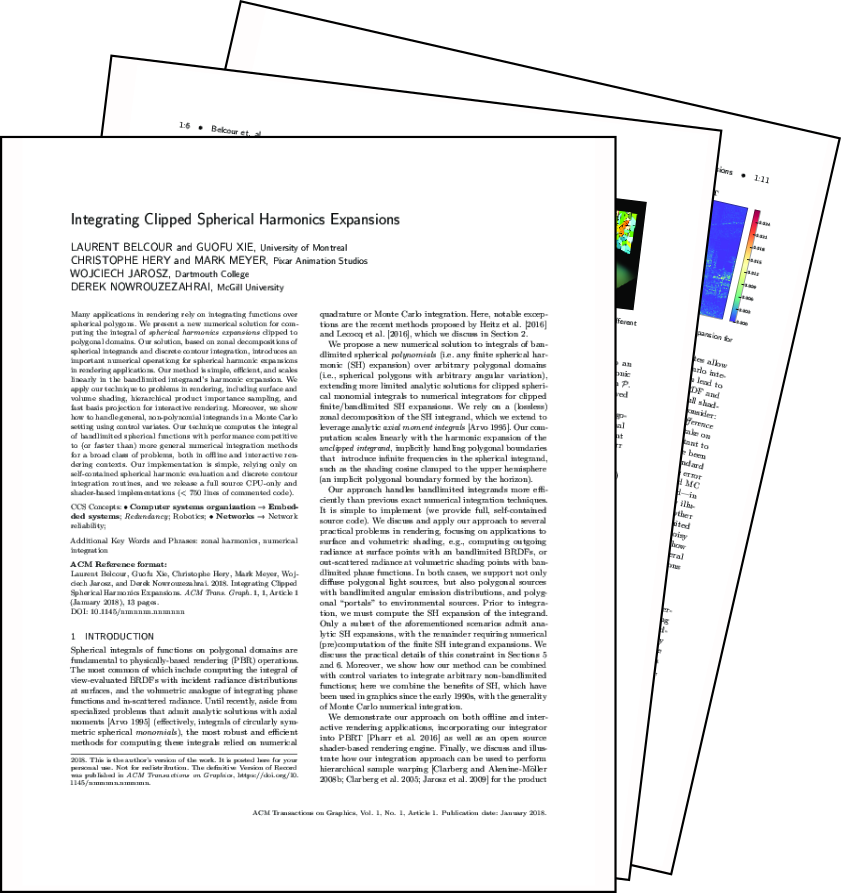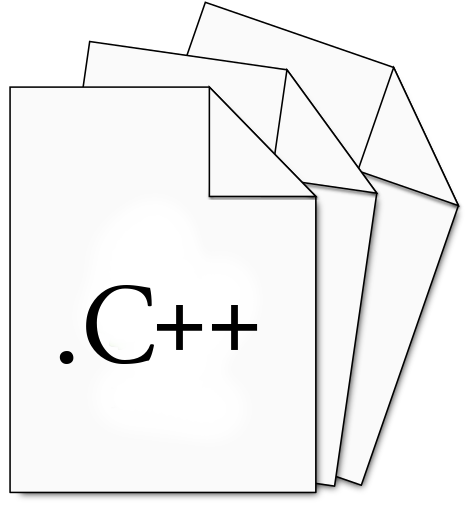paper code
available at belcour.github.io/blog